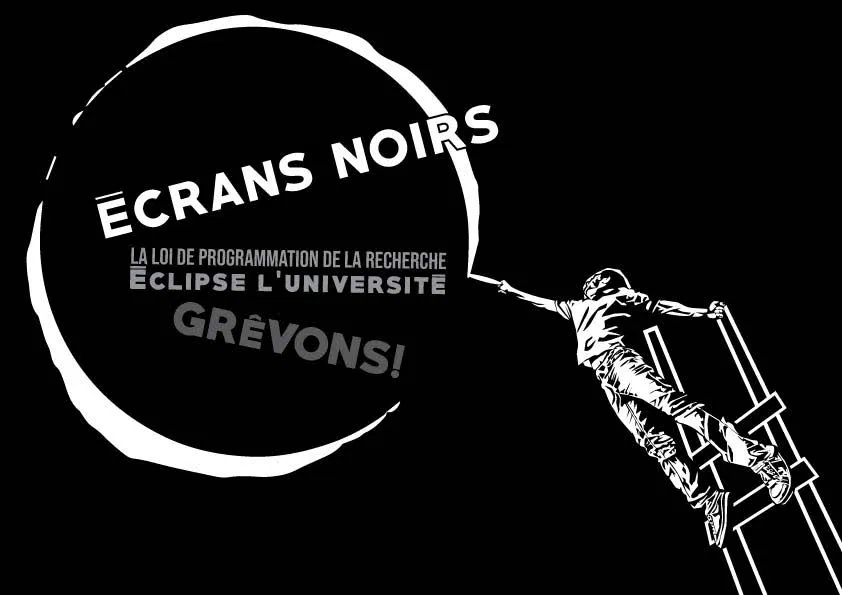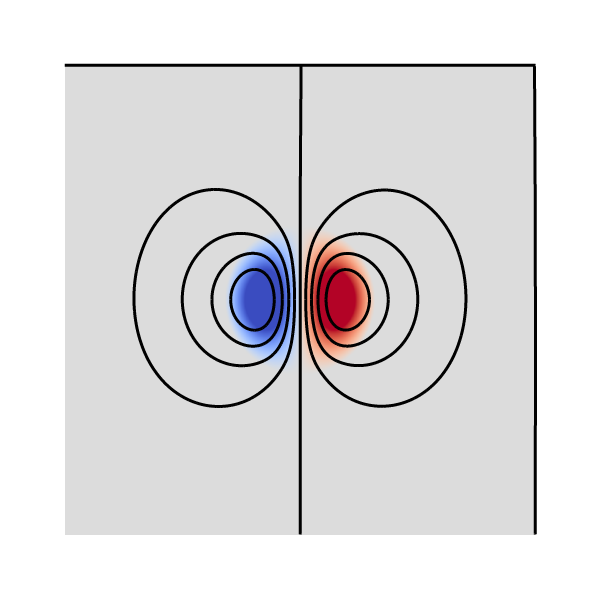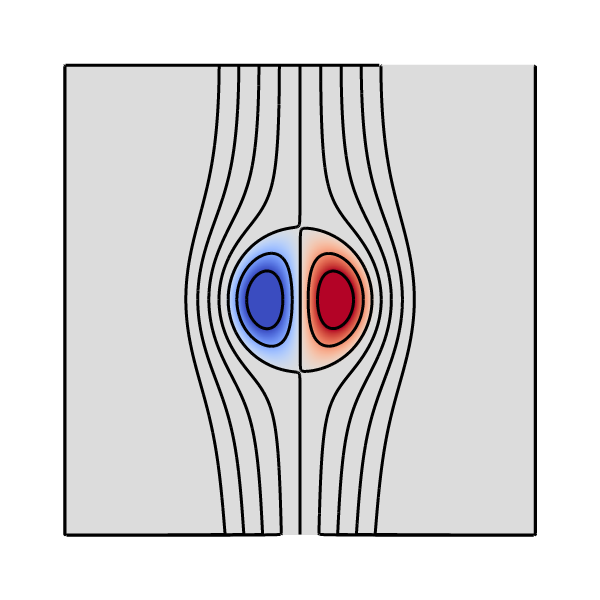# sandbox/Antoonvh/isoline.c

We plot an “isoline” for the stream function with Bview2D.

# include "view.h"
# include "poisson.h"
scalar omega[], psi[];

# include "fractions.h"
void iso_contour (scalar s, double isoval){
vertex scalar vs[];
scalar il[];
boundary ({s}); // Just in case ...
foreach_vertex()
vs[] = interpolate (s, x , y) - isoval;
boundary ({vs});
fractions (vs, il);
boundary ({il});
draw_vof("il", lw = 3);
}

A dipolar vortex structure is initialized according to a vorticity (\omega) distribution, and a corresponding stream function (\psi) is found by solving the associated Poisson problem.

int main(){
periodic(left);
psi[bottom] = dirichlet(0.); //The bottom boundary is a stream line
L0 = 2*pi;
X0 = Y0 = -L0/2;
init_grid (512);
double k = 3.83170597;
foreach(){
double r = pow(pow((x),2)+(pow((y),2)),0.5);
double s = (x)/r;
omega[] =  ((r<1)*((-2*j1(k*r)*s/(k*j0(k)))))*sq(k);
}
boundary ({omega});
poisson(psi, omega);
squares ("omega", map = cool_warm);
//isosurface ("s", 1); //<- Doesn't naively work in 2D

We plot a few isolines for \psi.

  for (double iv = -1; iv <= 1; iv += .25)
iso_contour(psi, iv);
save("result.png");
clear();

The resulting figure:Vorticity distribution and a few streamlines

We now realize that the stream function is not Gallilean invariant. We decide to study the streamlines in the frame that co-moves with the theoretical dipole.

  foreach()
psi[] += x;
squares ("omega", map = cool_warm);
for (double iv = -1; iv <= 1; iv += .25)
iso_contour(psi, iv);
save("result2.png");
}

the resulting file is called result2.png;Vorticity distribution and a few stream lines in the co-moving frame

It reveals that there exists as closed circular streamline around the two vortex structures. This means that fluid inside this so-called atmosphere will be entrained by the dipole.